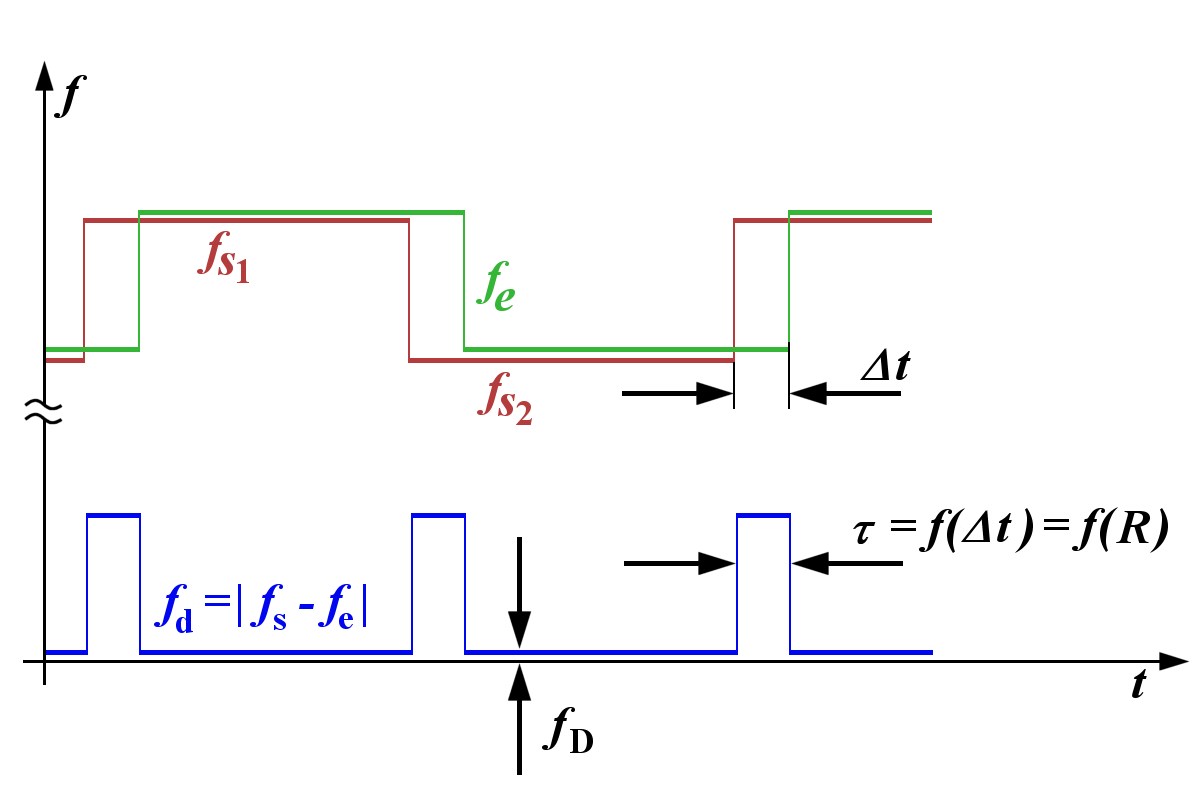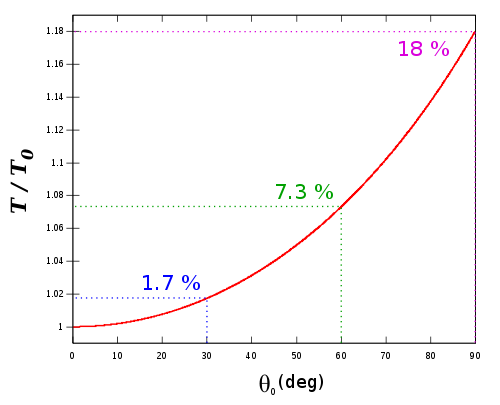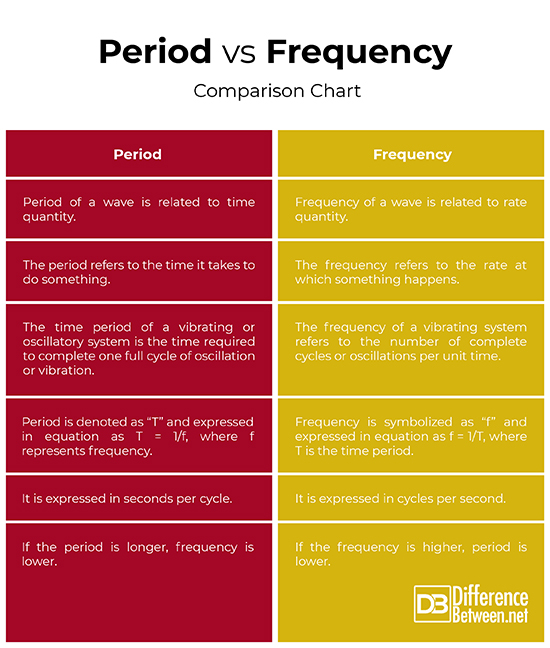#Difference Between Similar Terms and Objects

# Difference Between Period and Frequency

The vibrations and oscillations of mechanical systems remain one of the most important fields of study in physics. Virtually every system oscillates or vibrates freely in a large variety of ways.

What do an ocean buoy, a guitar, a child in a swing, or the beating of heart all have in common? They all oscillate – meaning they move back and forth between two points. The human body itself is a treasure-trove of vibratory phenomena. Even the atoms in our body vibrate. Every system that oscillates has something in common. It involves force and energy. You start a motion by pushing the child in a swing or you can increase the energy of atoms vibrating in a crystal with heat. So oscillations create waves.

What do oscillations or waves have in common? The feature that relates such phenomena is periodicity. It’s clear that a small number of underlying principles describe all of the phenomena which proves that they are common than you could have imagined. You’ll notice a certain pattern or movement in each phenomenon that repeats itself over and over again. A periodic motion is a motion that repeats itself over and over again at regular intervals, such as the one exhibited by a guitar string or the back and forth motion of a child in a swing. The time to complete one vibration cycle or oscillation is called the period of a wave. Frequency simply refers to the number of cycles of an oscillation occur per second.## What is Frequency?

Frequency of a wave simply refers to the number of complete cycles or oscillations that occur in one second. It is measured in cycles per second or hertz (Hz). A cycle is one complete oscillation and a vibration can be a single or multiple events, whereas oscillations are mostly repetitive for a number of cycles. It is usually denoted by the letter “f” and is expressed as:

f = 1/T, where ’T’ represents time period and ‘f’ is the frequency.## What is Period?

Period is the inverse of frequency and is defined as the time taken for one complete round trip of the vibration or oscillation. It simply refers to the time for something to occur periodically and it is measured in seconds per cycle. The time period is reciprocal of the frequency which means both the quantities are inversely proportional to each other. In equation form, period is expressed as:

T = 1/f, where ‘f’ is the frequency and ‘T’ is the time period.

## Difference between Period and Frequency

1. ### Definition of Period and Frequency

Both the terms period and frequency are related because they exhibit a certain pattern of movement, yet they are distinctly different. Both are related to periodic phenomenon and are often confused with each other. Period refers to the amount of time it takes a wave to complete one full cycle of oscillation or vibration. Frequency, on the contrary, refers to the number of complete cycles or oscillations occur per second. Period is a quantity related to time, whereas frequency is related to rate. Period simply refers to the time for something to occur periodically, whereas frequency means how often that happens.

1. ### Relationship of Period and Frequency

Both the quantities are inversely proportional to each other. The frequency is expressed in cycles per second, waver per second, vibrations per second, etc. and is usually denoted by the letter “f”. The period is expressed in seconds per cycle. The unit of frequency is Hertz (Hz) and “T” represents the time period of one complete oscillation.  In mathematical terms, both the quantities are reciprocal of each other. In equation form, frequency and period are expressed as:

f = 1/T, where f is frequency and T is period.

It can also be expressed as:

T = 1/f

1. ### Example of Period and Frequency

Let’s say a wave takes one second to oscillate up and down, which means the time period of the wave is 1 second. The frequency and period are inversely proportional to each other. Because only one cycle occurs in a second, frequency of the wave would be 1 cycle per second. And if the wave took half a second to oscillate the complete cycle, the period of that wave would be 0.5 seconds and the frequency would be 1/0.5 = 2, that is 2 cycles per second. So, longer the time period, lower the frequency and vice-versa.

### Period vs. Frequency: Comparison Chart## Summary of Period Vs. Frequency

Both frequency and time period are the fundamental parameters of waves, related to each other, yet they are distinctly different in terms of quantity. The frequency of a wave is related to rate quantity, whereas the time period of a wave is related to time quantity. The time period refers to the time it takes for a wave to complete one full cycle of oscillation or vibration, which is inverse of the frequency. The frequency refers to the number of vibrations that occur in one second, which is the inverse of time period. Frequency is usually measured in Hertz (Hz) and period is measured in seconds. Both are inversely proportional to each other, which means if the frequency is higher, then the period is lower, and vice-versa. Frequency is denoted by the letter ‘f’, and period is denoted by ‘T’.

### Search DifferenceBetween.net :

Custom Search

Help us improve. Rate this post!Loading...Email This Post : If you like this article or our site. Please spread the word. Share it with your friends/family.

Please note: comment moderation is enabled and may delay your comment. There is no need to resubmit your comment.

## References :

Image credit: https://en.wikipedia.org/wiki/Frequency-shift_keying#/media/File:FSK-FMCW_Principle.png